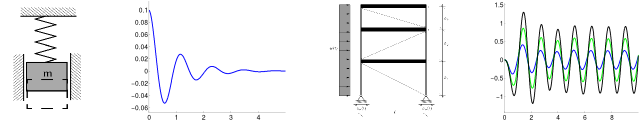# Finite Element Method

Typical models in engineering are defined by differential equations. It is often impossible to provide exact solutions to these models, so that they may only by approximated by numerical methods (simulation methods) using computers. The finite element method (FEM) is the most important method in structural analysis. Essential steps are the discretization of the domain of interest by means of elements and nodes, the assembly and solution of a system of equations for the solution at the nodes, and the visualization and interpretation of the results. In this course, several models in one, two, and three dimensions are introduced, reformulated for the use of FEM, and implemented in Matlab®. Important properties of the solutions and error sources in the approximations are discussed.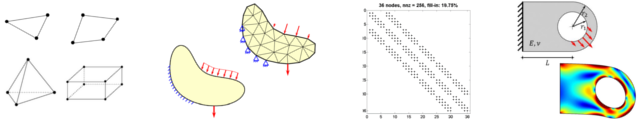# Nonlinear Finite Element Methods

The focus of this course is on non-linear models and their approximation with the finite element method (FEM). There are two major sources for non-linearities: geometric non-linearities have to be considered for large deformations, where the equilibrium has to be fulfilled in the deformed configuration (in contrast to linear analysis where equilibrium is fulfilled in the undeformed configuration). Material non-linearities are present when the material behaves in a plastic and/or viscous manner. The FEM for non-linear models yields non-linear systems of equations which have to be solved iteratively, e.g. by the Newton-Raphson method.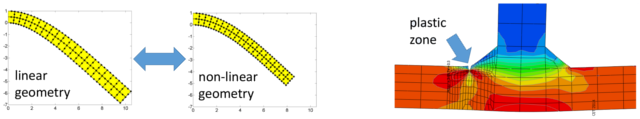# Numerical Methods in Structural Analysis

Various selected topics in computational structure analysis are addressed in this research seminar. Brevity and comprehensibility are emphasized and half of the time is reserved for discussions. In addition, doctoral students get the opportunity to present selected topics of their work which are also of general interest for graduate students in engineering.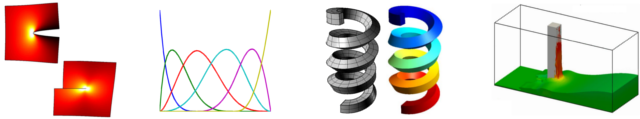# Boundary Element Methods

The boundary element method (BEM) is a numerical method which requires only a boundary discretization of the domain of interest. Hence, no mesh in the domain is needed resulting in fewer degrees of freedom than in the FEM. Furthermore, the BEM is able to handle (semi-)infinite domains, which is useful in the simulation of foundations and in tunneling for example. The course covers several models and their reformulation for the use in BEM. The assembly of the system of equations is detailed, solutions are verified, and practical applications are considered. The implementation of the BEM is demonstrated in Matlab®.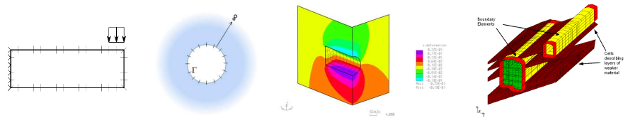# Structural Dynamics

This course focuses on the influence of dynamical loading on structures. Starting point is a simple mass oscillator, the resulting model equations, their analytical solution, and the interpretation of the results. Next, systems of oscillating masses are considered and numerical solution methods such as the Newmark scheme are introduced. Finally, typical engineering structures are considered in order to develop an understanding of the interplay between dynamic loading and the movement of structures. Resonance phenomena and the influence of damping is demonstrated.